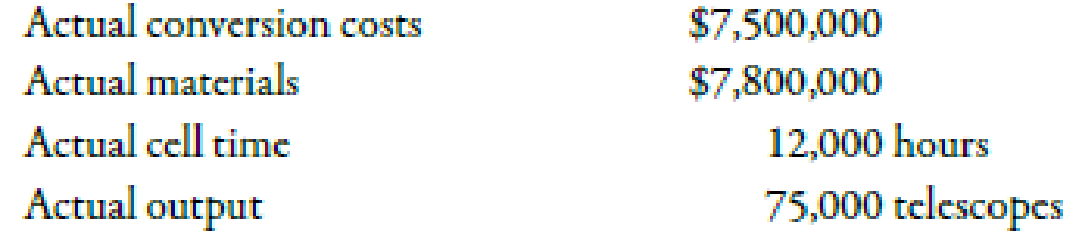Chapter 5, Problem 62P### Managerial Accounting: The Corners...

7th Edition
Maryanne M. Mowen + 2 others
ISBN: 9781337115773

#### Solutions

Chapter
Section### Managerial Accounting: The Corners...

7th Edition
Maryanne M. Mowen + 2 others
ISBN: 9781337115773
Textbook Problem
5 views

# Cycle Time, Velocity, Product CostingGoldman Company has a JIT system in place. Each manufacturing cell is dedicated to the production of a single product or major subassembly. One cell, dedicated to the production of telescopes, has four operations: machining, finishing, assembly, and qualifying (testing).For the coming year, the telescope cell has the following budgeted costs and cell time (both at theoretical capacity):During the year, the following actual results were obtained:Required:(Note: Round answers to two decimal places.) 1. Compute the velocity (number of telescopes per hour) that the cell can theoretically achieve. Now, compute the theoretical cycle time (number of hours or minutes per telescope) that it takes to produce one telescope. 2. Compute the actual velocity and the actual cycle time. 3. CONCEPTUAL CONNECTION Compute the budgeted conversion costs per minute. Using this rate, compute the conversion costs per telescope if theoretical output is achieved. Using this measure, compute the conversion costs per telescope for actual output. Does this product-costing approach provide an incentive for the cell manager to reduce cycle time? Explain.

1.

To determine

Calculate the amount of theoretical velocity and theoretical cycle time.

Explanation

Cycle Time:

Cycle time is the activity performance measure that calculates the duration of time which is required to manufacture one unit of product.

Velocity refers to the number of units that is manufactured in the given duration of time. For example, output per hour.

Use the following formula to calculate the theoretical velocity:

TheoreticalVelocity=Theoretical OutputCell Time

Substitute 90,000 telescopes for theoretical output and 12,000 hours for cell time in the above formula.

Velocity=90,00012,000hours=7

2.

To determine

Calculate the value of actual velocity and actual cycle time.

3.

To determine

Calculate the value of budgeted conversion costs, theoretical conversion costs and actual conversion costs. Identify whether a product costing approach helps in reducing the cycle time.

### Still sussing out bartleby?

Check out a sample textbook solution.

See a sample solution

#### The Solution to Your Study Problems

Bartleby provides explanations to thousands of textbook problems written by our experts, many with advanced degrees!

Get Started

#### What does recognition mean in accounting?

Intermediate Accounting: Reporting And Analysis

#### WACC Midwest Electric Company (MKC) uses only debt and common equity. It can borrow unlimited amounts at an int...

Fundamentals of Financial Management, Concise Edition (with Thomson ONE - Business School Edition, 1 term (6 months) Printed Access Card) (MindTap Course List)

#### Give an example of a future cost that is not relevant.

Cornerstones of Cost Management (Cornerstones Series)